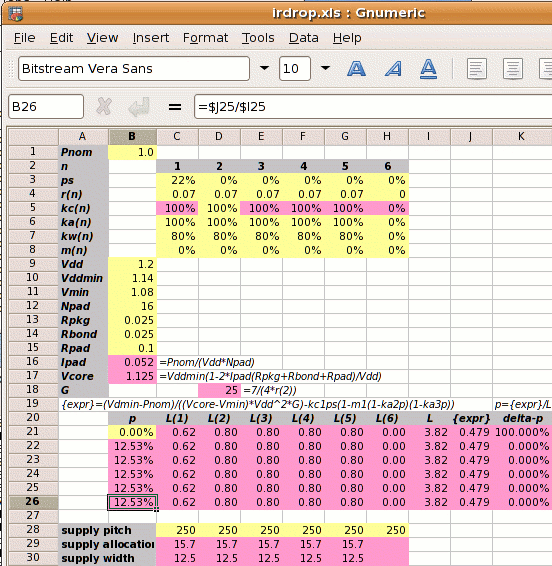The power strap calculation follows 5 steps.

Step 1: Calculate Ipad and Vcore:

1⁄(1.2×16) = 0.052A
Vcore =
1.14×(1−2×0.052×(0.025+0.025+0.1)⁄1.2
1.125V

Step 2: Calculate the reference power supply conductance G:

G =
 7 4×r2

7 ⁄ (4 × 0.07) =  25 mhos

Step 3 is to set out the values of kan, kwn, kcn and mn for each metal layer, and use these to calculate the value of L.

metal layer 1 2 3 4 5
kan 100% 100% 100% 100% 100%
power metal allocated coefficient
kwn  80%  80%  80%  80%  80%
power metal used coefficient
kcn 100% 100% 100% 100% 100%
conductivity coefficient
mn   0%   0%   0%   0%   0%
core area blocked
kan and kcn are 100% because all power straps have the same space allocated and the metal resistivities are the same. kwn is 80% for all metal layers because the power strap widths and spacings are also all the same.

Normally we can't calculate L directly because its value depends on p which we don't know. But in this case with m1‑5=0 we can

 L = kw1kc1(1-ps)(1-m1(1-ka2p)(1-ka3p))+ kw2kc2(1-m2(1-ka2p)(1-ka3p))+ kw3kc3(1-m3(1-ka2p)(1-ka3p))+ kw4kc4(1-m4(1-ka2p)(1-ka3p))+ kw5kc5(1-m5(1-ka2p)(1-ka3p)) = ( 0.62 + 0.8 + 0.8 + 0.8 + 0.8 ) = 3.82

Step 4: Calculate the power strap allocation percentage p:

p =
 { Vddmin×Pnom −kc1×ps } × 1 (Vcore−Vmin)×Vdd2×G L
 { 1.14×1 −1×0.22 } × 1 (1.125−1.08)×1.22×25 3.82
(0.701−0.222)×0.262 = 12.53%

As shown on the right, a spreadsheet can be used to iterate to the answer. Yellow squares are user input; pink squares are calculated values.

Since in this example all the kan and kwn are equal, the strap allocation and widths are the same for each layer.
If we decide to set the power strap pitch to 250µm, then each Vdd and Vss supply strap width Wsn is:

 Wsn = 250 × p × kw ⁄ 2 =250 × 0.1253 ×0.8 ⁄ 2 = 12.5µm

Step 5: Calculate the new core size. If the initial core size estimate without power straps is x, then with power straps the core size becomes x

 x′ = x = x = x = x+14.33% √(((1-ka2p)(1-ka3p)) √0.87472 0.8747

The value 14.33% is called the IR Drop Adder.

1W is not a very high power consumption, yet 12.5% of each metal layer must be allocated to extra power straps for the required power, and the die side increased by 14.3%.

Design Attribute Value
Pnom core power consumption 1W
ps fraction of metal-1 in the standard cells used for power supplies 22% (for vsclib)
rn resistivity of metal layer n in ohms per square 0.07Ω per sq.
kan
 user defined   ratio of metal layer n allocated to power metal-2 allocated to power
100%
kwn
 user defined   ratio of metal layer n used for power metal-2 allocated to power
80%
mn percentage of metal layer n blocked to power straps 0%
Vdd the nominal supply voltage 1.2V
Vddmin the minimum supply voltage, 5% less than nominal 1.14V
Vmin the desired voltage at the centre of the die, 10% less than the nominal 1.08V
Rpkg the resistance of the package leadframe 25mΩ
Rbond the resistance of the bond wire 25mΩ